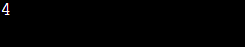# Count of groups of consecutive 1s in a given Binary String

## Question

You are given a Binary String S. Return the number of groups in S, containing 1s only.

Input:    A string S, and an integer N, representing the length of S.
Output: An integer returning the count of groups in S containing only 1s.

Alert Ninjas:  Don’t stop now! Enroll in the Competitive Programming course today and start coding. Learn the art of writing time and space-efficient code and compete in code competitions worldwide.

Example:
Input: S = 1010011101,  N = 10
Output: 4

Explanation:

The following four groups are of 1s only:

1. The group over the range [0, 0] is equal to “1”.
2. The group over the range [2, 2] is equal to “1”.
3. The group over the range [5, 7] is equal to “111”.
4. The group over the range [9, 9] is equal to “1”.

## Solution

Recommended: Please try to solve it yourself first before moving on to the solution.

The idea is simple: We will solve it by simply iterating over the string and maintaining a stack for the group of 1s.

To solve the problem, follow the steps mentioned below:

• Initialize a variable count as 0 to keep track of the number of substrings of 1s in S.
• To store the substring of 1s, create a stack called st.
• Using the variable i, iterate over the characters of the string S and perform the following:
• Push 1 onto stack st if the current character is 1.
• Otherwise, if st isn't empty, add 1 to count. Else Clear st.
• If st is not empty, increase count by one, i.e., if a suffix of 1s exists.
• Return count.

C++  code

OutputTime Complexity: O(N)

Auxiliary Space: O(N)

## Key takeaways

In this article, we solved an array question using stacks. We counted the number of groups in the array that contained only 1s.